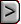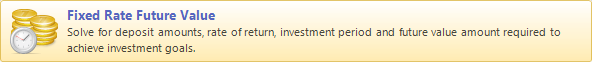﻿ Fixed Rate Future Value
WinAmort Professional 2.0

Fixed Rate Future ValueFixed Rate Future ValueFixed Rate Future Value“If a man has money, it is usually a sign too that he knows how to take care of

it: don’t imagine his money is easy to get simply because he has plenty of it.”

E. Watson Howe, 1911-

 How much would accumulate if you were to place \$100 in the bank every month at 9% interest paid annually? This is the type of question that represents the essence of future value calculations.   months             12 x 12 x 12 x 12 x 12 x 12 x 12 x 12 x 12 x 12 x                         \$     \$      \$      \$     \$      \$     \$     \$      \$     \$                       100  100  100  100  100  100  100  100  100  100 year                   1      2      3     4      5      6     7     8      9    10   The above diagram graphically illustrates deposits of \$100 every month into a bank account paying “x” percent interest and continuing for 10 years. How much would accumulate at the end of the 10th year? To be more specific let’s assume the interest rate is 10% per year, compounded annually. At the end of the first year you would accrue \$1200 plus you would get 10% interest on the \$1200. Therefore we would have \$1200 + \$120 = \$1320 at the end of the first year.   The next year you would add another \$1200 dollars to the \$1320. Thus accumulating \$2520 on which 10% interest would be calculated again. This would give you \$2520 plus another \$252 this would bring your total \$2772 in savings.  If this continued for another 8 years, you would have a grand total of \$20484.50 at the end of the 10th year. This value is the future value of investing \$100 every month for 10 years at an interest rate of 10% compounded annually. If \$1200 had been deposited without interest, every year for 10 years, there would only be \$12,000 accumulated at the end of the 10th year. This type of calculation can be helpful when one needs to know how much can be saved by making regular periodic deposits of a certain amount. In other words setting up an annuity.